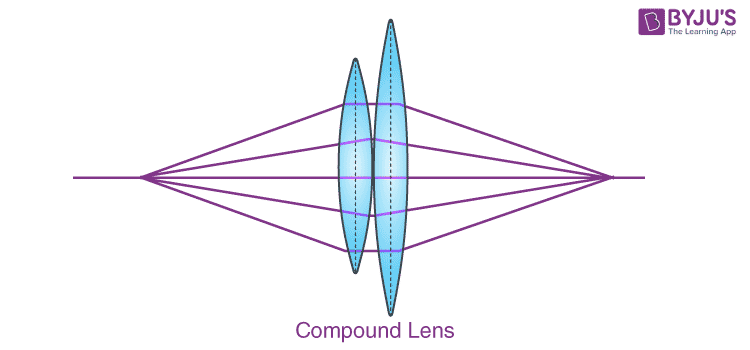# Compound Lenses - Thin Lenses In Contact

A simple lens system consists of the use of only one lens as opposed to the compound lens system where multiple lenses can be used with a single common axis. The simplest of these compound lens systems are two thin lenses kept in contact with each other. Let’s study compound lenses and their properties in this article.

Commonly Used Lenses are:

1. Convex (Converging lens)
2. Concave (Diverging lens)

As you know, we have discussed in detail the convex lens and concave lens in our previous sessions. Now, let us learn about compound lenses.

## Compound Lenses

The depiction of the compound lens is shown in the figure below. Compound lenses are the type of lenses that features two thin lenses that are mounted on a common axis usually closer to each other or often cemented together.The common focal length for a system, where two thin lenses sharing an axis are kept in contact with each other, is given by the following formula.

$$\begin{array}{l}\frac{1}{f} = \frac{1}{f_1} + \frac{1}{f_2}\end{array}$$

where,

f is the combined focal length

f1 is the focal length of the first lens

f2 is the focal length of the second lens

Since the power is the reciprocal of the focal length, what is very evident in this case? For thin lenses in contact, it is pretty clear that the combined power of the system is given by adding the powers of the individual lenses.

But what if the lenses aren’t in contact with each other? If the lenses are separated by a distance ‘d’, then, in this case, the combined focal length can be calculated using the following formula.

$$\begin{array}{l}\frac{1}{f} = \frac{1}{f_1} + \frac{1}{f_2} – \frac{d}{f_1.f_2}\end{array}$$

Why don’t you try to solve the following simple problem to see if you understand the concept? A bi-convex lens of focal length = 10 cm and a bi-concave lens of focal length = 20 are kept in contact with each other. Both of these have the same value of the refractive index. Find the combined focal length.

While dealing with the combination of lenses or compound lenses, you may come across the following terms.

### A Focal System

When the distance of separation between the two lenses (d) is equal to the sum of the individual focal lengths (f1 + f2), the combined focal length is infinite. This makes the refracted light rays parallel to each other.

### Achromatic Doublet

The lenses used are combined in such a way that two wavelengths (typically the ends red and violet) are brought into focus. This is one of the applications of using a compound lens system, where chromatic aberrations (which can be found using single lenses) are corrected.

To know about lens formula, magnification and power in detail, see the video below.### Application of Compound Lenses

We find compounded lenses used in telescopes and microscopes where two or more lenses are combined in order to:

• Reduce defects caused by using a single lens.
• Get an erect image of an object.
• Increase the magnification of the image.

Hope you have understood about compound lenses. To know more about various types of lenses and learn about ray optics with our expert faculty, visit BYJU’s – The Learning App.

## Frequently Asked Questions – FAQs

Q1

TRUE
Q2

### What are the common types of lenses?

The following are the types of lenses:

• Convex lens
• Concave lens
Q3

### Define a convex lens?

It is a type of lens that is thicker at the centre and gets thinner towards the edges. The convex lens converges into a straight beam of light.

Q4

### Give the formula to find the common focal length for a system, where two thin lenses sharing an axis are kept in contact with each other.

$$\begin{array}{l}\frac{1}{f} = \frac{1}{f_1} + \frac{1}{f_2}\end{array}$$
Q5

### When the distance of separation between the two lenses (d) is equal to the sum of the individual focal lengths (f1 + f2), the combined focal length is _________

Infinite

Test Your Knowledge On Compound Lenses Thin Lenses In Contact!